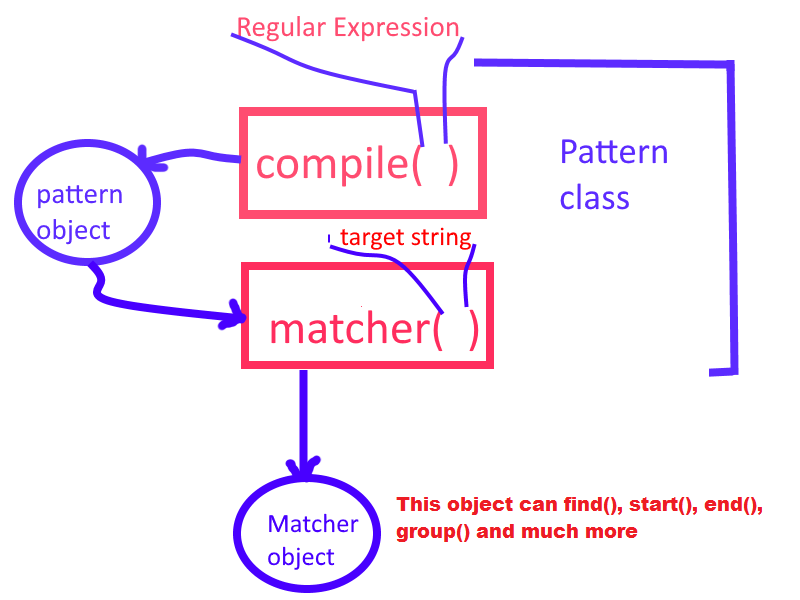Home ProgrammingJava Regular Expressions in java

# Regular Expressions in java

0 comment 530 views

In general regular expressions represent patterns. Similarly in java, Regular expressions represent group of string objects with a particular pattern.

### Applications of Regular Expression:-

• Validation
• Pattern matching applications
• Translator designing (compiler, interpreter)
• Lexical analysis
• Digital circuit Designing
• Communication protocol developments

Generally we’ll see the problems in which we have a string and a pattern. Then wee need to check whether the string match the pattern or not. This is clear that we need a string and a pattern, then we can use regular expression. Simply we can work in simple pattern matching application while learning.

Regular expressions are introduced in 1.4 version of java.  A pattern object represent compiled version of the regular expression. We can say pattern is equivalent java object of regular expression. All the classes related to regular expressions are contained in java.util.regex package.

### Creating Pattern object:-

We create pattern object by calling static factory method of class Pattern, compile(). Static means we call by using class name and factory means it return object of same class.

Signature of compile():

`public static Pattern compile(String regularExpression)`

For Example:

`10. Pattern p = Pattern.compile(".xy");`

This statement will create a pattern object for given regular expression.

### Creating Matcher object:-

We need a Matcher object to perform matching operations. Matcher object is used to match pattern into target string. To create Matcher object we use matcher() method of Pattern class.

Signature of matcher():

`public Matcher matcher(String targetString)`

For Example:

`11. Matcher m = p.matcher("xxyy1xyxyyWxyP");`

This statement will create a matcher object for target string.### Some Important methods of Matcher class:

• public boolean find() // This method return true if it find next match
• public int start() // this method will return index of match
• public int end() // this will return last index of match + 1
• public String group() // This will return the sub-string of target string which matches.

### Illustrate everything by example:-

```import java.util.regex.Matcher;
import java.util.regex.Pattern;

class RegExample{

public static void main(String args[]){

Pattern p = Pattern.compile("xy"); //
Matcher m = p.matcher("xxyy1xyxyyWxyP");
while(m.find()){

System.out.println( m.group()+" matches from "+m.start()+" to "+m.end());
}

}

}```

Here you can imagine output. Matches are bold.

 x x y y 1 x y x y y W x y P 0 1 2 3 4 5 6 7 8 9 10 11 12 13

### Output:Thanks..

0 comment